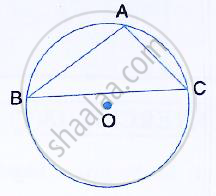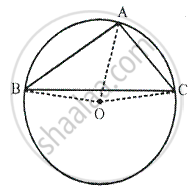Share

The Figure Shows a Circle with Centre O. Ab is the Side of Regular Pentagon and Ac is the Side of Regular Hexagon. Find the Angles of Triangle Abc. - ICSE Class 10 - Mathematics

ConceptArc and Chord Properties - the Angle that an Arc of a Circle Subtends at the Center is Double that Which It Subtends at Any Point on the Remaining Part of the Circle

Question

The figure shows a circle with centre O. AB is the side of regular pentagon and AC is the side of regular hexagon.
Find the angles of triangle ABC.SolutionJoin OA, OB and OC
Since AB is the side of a regular pentagon,
∠AOB = (360°)/5 = 72°
Again AC is the side of a regular hexagon,
∠AOC = (360°)/6 = 60°
But ∠AOB + ∠AOC + ∠BOC = 360° [Angles at a point]
⇒ 72° + 60° + ∠BOC = 360°
⇒ 132° + ∠BOC = 360°
⇒ ∠BOC = 360° -132°
⇒ ∠BOC = 228°
Now, Arc BC subtends ∠BOC at the centre and
∠BAC at the remaining part of the circle.

⇒ ∠BAC = 1/2 ∠BOC

⇒∠BAC = 1/2xx 228° = 114°
Similarly, we can prove that
⇒∠ABC = 1 /2∠AOC
⇒∠ABC = 1 /2 xx 60° = 30°  And
⇒∠ACB = 1/2 AOB
⇒ ∠ACB = 1/2 xx  72° = 36°
Thus, angles of the triangle are, 114°, 30° and 36°

Is there an error in this question or solution?

Video TutorialsVIEW ALL 

Solution The Figure Shows a Circle with Centre O. Ab is the Side of Regular Pentagon and Ac is the Side of Regular Hexagon. Find the Angles of Triangle Abc. Concept: Arc and Chord Properties - the Angle that an Arc of a Circle Subtends at the Center is Double that Which It Subtends at Any Point on the Remaining Part of the Circle.
S